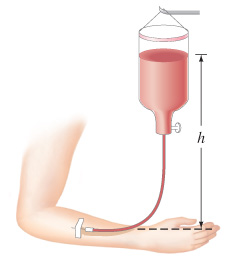Problem: Intravenous infusions are often made under gravity, as shown in the figure.a) Assuming the fluid has a density of 1.00 g/cm3, at what height h1 should the bottle be placed so the liquid pressure is 58 mm-Hg ?b) At what height h2 should the bottle be placed so the liquid pressure is 660 mm-H2O ?c) If the blood pressure is 78 mm-Hg above atmospheric pressure, how high should the bottle be placed so that the fluid just barely enters the vein?

Problem Details

Intravenous infusions are often made under gravity, as shown in the figure.
a) Assuming the fluid has a density of 1.00 g/cm3, at what height h1 should the bottle be placed so the liquid pressure is 58 mm-Hg ?
b) At what height h2 should the bottle be placed so the liquid pressure is 660 mm-H2O ?
c) If the blood pressure is 78 mm-Hg above atmospheric pressure, how high should the bottle be placed so that the fluid just barely enters the vein?